International
Tables for
Crystallography
Volume C
Mathematical, physical and chemical tables
Edited by E. Prince

International Tables for Crystallography (2006). Vol. C, ch. 7.3, p. 644

## Section 7.3.2. Neutron capture

P. Converta and P. Chieuxa

aInstitut Laue–Langevin, Avenue des Martyrs, BP 156X, F-38042 Grenoble CEDEX, France

### 7.3.2. Neutron capture

| top | pdf |

Neutrons' lack of charge and the fact that they are only weakly absorbed by most materials require specific nuclear reactions to capture them and convert them into detectable secondary particles. Table 7.3.2.1lists the neutron-capture reactions that are commonly used in thermal neutron detection. The incoming thermal neutron brings a negligible energy to the nuclear reaction, and the secondary charged particles or fission fragments are emitted in random directions following the conservation-of-momentum law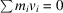. The capture or absorption cross sections for a number of nuclei of interest are plotted as a function of neutron energy in Fig. 7.3.2.1.These cross sections are commonly expressed in barns (1 barn = 10−28 m2). At low energies, they are inversely proportional to neutron velocity, except in the case of Gd, which has a nuclear resonance at 0.031 eV. The total efficiencyof neutron detection can be expressed by the equation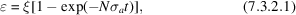where N is the number of absorbing nuclei per unit volume,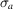is their energy-dependent absorption cross section, and t is the thickness of the absorbing material. The factor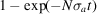gives the neutron-capture efficiency, while ξ is a factor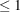that depends on the detector geometry and materials (absorption and scattering in the front window) and on the efficiency of the secondary particles.

 Table 7.3.2.1| top | pdf | Neutron capture reactions used in neutron detection
 n = neutron, p = H+ = proton, t = 3H− = triton, α = 4H+ = α, alpha, e− = electron.
Capture reactionCross section at 1 Å (barns)Secondary-particle energies (MeV)
3He + nt + p 3000 t  0.20 p  0.57
6Li + nt + α 520 t  3.74 α  2.052100

α  1.47
α  1.78

7Li  0.83
7Li  1.01

γ  0.4874000
(natGd: 17000)
e spectrum
γ spectrum
0.07 to 0.182
up to 8

235U + n → fission fragments 320 Fission fragments up to 80Figure 7.3.2.1 | top | pdf |The capture cross sections for a number of nuclei used in neutron detection. [Adapted from Convert & Forsyth (1983).]

### References

Convert, P. & Forsyth, J. B. (1983). Position-sensitive detection of thermal neutrons: Part 1, Introduction. Position-sensitive detection of thermal neutrons, pp. 1–90. London: Academic Press.